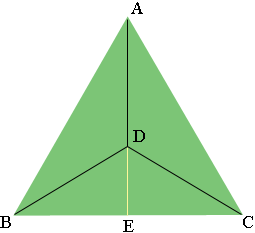SEARCH HOMEMath Central Quandaries & QueriesQuestion from BIMAL, a parent: what is the diameter of a circle drawn around a equilateral triangle of size 6 cmHi,

In my diagram $D$ is the center of the circle.If the circle passes through the three vertices $A, B$ and $C$ then the lengths $|AD|, |BD|$ and $|CD|$ are equal. What is the measure of the angle $BCD?$

$DE$ is an extension of $AD.$ What is the length of $EC?$ What is the length of $CD?$

PennyMath Central is supported by the University of Regina and The Pacific Institute for the Mathematical Sciences.